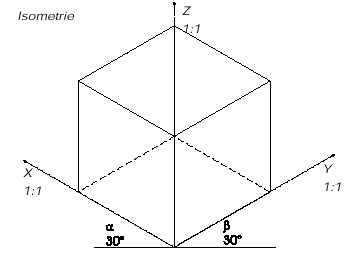Версия:  Allplan 2017 / Allplan 2016 / Allplan 2015  | Последнее изменение: 26.04.2017
Not voted yet. Be the first to vote this FAQ as helpful!

Question:

When I create a hidden line image of an isometric view (Viewmenu -> View-> Front Left, Southwest Isometric Viewor any other isometric view), Allplan does not use the original dimensions so that the resulting lengths are distorted.
How can I make sure that Allplan creates the hidden line image based on the actual dimensions?

Background information:

In accordance with DIN, an isometric view is calculated as follows:
Allplan chooses the projection plane and the axes so that all the axes are shortened equally = axonometric projection of an isometric view.
This is the case when all the angles are the same: 120 degrees. The result is an equilateral triangle. Each side is shortened by two-thirds or 0.8165. However, the edges parallel to the axes are usually not shortened.

Isometric view:Angle of the coordinate axes:
x axis: 30 degrees to the horizontal
y axis: 30 degrees to the horizontal

Shortening:
x : y : z = 1 : 1 : 1 (using a factor of 0.8165)

Solution:

When calculating an isometric view, Allplan shortens all directions by the same value (x:y:z = 1:1:1), using a factor of 0.8165.
All you need to do is resize the result in the destination drawing file by the corresponding factor (1.22475) in the x and y directions. After this, all the edges are displayed at their actual size and can be dimensioned.

Загрузка PDF  FAQ_EN_Hidden_line_image_creating_an_isometric_view_...